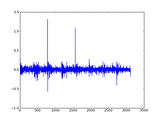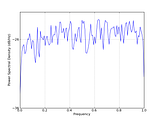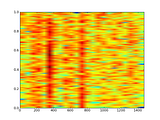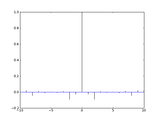# Daily and weekly cycles of the Dow Jones

This entry is part of 15 in the series Grails Finance

Grails Finance 1.3

The Elliott Wave Principle posits that there are at least 5 waves to be reckoned with. NBER has its own definition of business cycles, which are measured in months. I had a look at daily and weekly historical data with the help of the Page distribution.

## Page distribution

The page distribution takes the Fourier transform of a signal, squares the absolute value of that and then differentiates it in respect to time. I simplified this a bit and wrote the following Java code

```1 2 3 4 5 6 7 8 9 10 11 12 13 14 15 16 17 18 19 20 21 22 23 24 25 26 27 28 29 30 31 32 33 34 35 36 37 38 39 40 41 42 43 44 45 46 47 48 49 50 ... public TreeMap<Double, Integer> page(double[] xt) { Complex[] transform = new FastFourierTransformer().transform(xt); TreeMap<Double, Integer> map = new TreeMap<Double, Integer>();   double[] amps = amplitude(xt, transform);     for(int i = 2; i < amps.length; i++) { map.put(amps[i], i); }   return map; }   public List<Integer> top9(double[] xt) { List<Integer> list = new ArrayList<Integer>(); TreeMap<Double, Integer> map = page(xt);   for(int i = 0; i < 9; i++) { list.add(map.pollLastEntry().getValue()); }   return list; }   private double[] amplitude(double[] xt, Complex[] transform ) { double[] amps = new double[transform.length];   for (int i = 1; i < transform.length; i++) { double doubleReal = calcFormula(xt, transform, i); int period = Math.round(xt.length/ (float) i);   if(period < amps.length) amps[period] += Math.abs(doubleReal); }   return amps; }   private double calcFormula(double[] xt, Complex[] transform, int i) { Complex conjugated = transform[i].conjugate().multiply(xt[i]); double phi = Math.atan(-2 * Math.PI * i); Complex ejpt = new Complex(Math.cos(phi), Math.sin(phi)); conjugated = conjugated.multiply(ejpt); double doubleReal = 2 * conjugated.getReal();   return doubleReal; } ...```

and unit tests

```1 2 3 4 5 6 7 8 9 10 11 12 13 14 15 16 17 18 19 20 21 ... public double f(double x) { return 7 + Math.sin(2 * Math.PI * x / 23) - Math.cos(2 * Math.PI * x / 42) + Math.sin(2 * Math.PI * x / 7); }   @Test public void testPage() { double[] doubles = new double;   for (int i = 0; i < doubles.length; i++) { doubles[i] = f(i); }   TreeMap<Double, Integer> map = new PageDistribution().page(doubles); assertEquals(23, map.pollLastEntry().getValue().intValue()); assertEquals(42, map.pollLastEntry().getValue().intValue()); assertEquals(7, map.pollLastEntry().getValue().intValue()); } ...```

## Weekly data extraction

I extract weekly data by taking out all the fridays in the dataset. Hopefully there are not too many holidays that fall on a friday :). Jodatime has some facilities for friday selection.

```1 2 3 4 5 6 7 8 9 10 11 12 13 14 ... def queryWeeklyVals(symbol,fieldName) { def instrument = Instrument.findBySymbol(symbol) def field = Field.findByName(fieldName) def fieldVals = FieldValue.findAllByInstrumentAndField(instrument, field) fieldVals = fieldVals.findAll { it.added.getDayOfWeek() == DateTimeConstants.FRIDAY } fieldVals = fieldVals.sort { a, b -> a.added <=> b.added } def data = []   fieldVals.each { data << Double.valueOf( it.val )}   return data } ...```

## Weighted moving average

It seemed like a good idea to plot the weighted moving average of the dataset.

```1 2 3 4 5 6 7 8 9 10 11 12 13 14 15 16 17 18 19 20 21 22 23 24 25 26 ... def wma(vals, period) { def averages = []   for( i in (period - 1)..<vals.size()) { def sublist = vals[i..< Math.min(period + i, vals.size() )] averages << weightedMean(sublist as double[]) }   return averages }   def weightedMean(vals) { def total = 0.0 def weightsSum = 0.0   for(i in 0..<vals.size()) { if(vals[i] == null) vals[i] = 0.0   total += i * vals[i] weightsSum += i }   return total/weightsSum } ...```

So there we have it, lots of waves just as the Elliot Wave Principle requires, but unfortunately not conforming to the NBER business cycles. As promised here is the spreadsheet for the daily and weekly relative changes of the close price.

I installed the Scipy superpack, which contains amongst others Matplotlib, gFortran and NumPy.

```1 ipython -pylab```

starts an interactive mathematical environment. I loaded 1 minute bars IBM intraday data from 29 september till now.

```1 c,v = loadtxt('IBM_1.csv', delimiter=',', usecols=(6,7), unpack=True)```

and made a plot of the ‘close’ price histogram. The close price is I guess the last price observed within the one minute window.

```1 hist(c, 100)```

If I did not know better, I would say that there are several gaussians to be seen.

Plotting the prices against time requires a few extra lines.

```1 2 3 4 t = arange(0.0, 3120.0, 1) ax1 = subplot(211) plot(t, c) show()```

With a small modification, I can plot log values on the y axis.

```1 2 3 t = arange(0.0, 3120.0, 1) plt.semilogy(t, c) show()```

Almost a straight line?

If you can live with the default settings, plotting the power spectral density and spectogram are oneliners.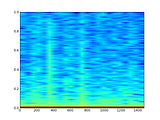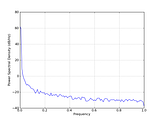It’s just as easy to plot differences, PSD, spectogram and autocorrelation.

```1 2 3 4 5 6 t = arange(1.0, 3120.0, 1) d = diff(c) plot(t, d) psd(d) specgram(d) acorr(d)```